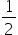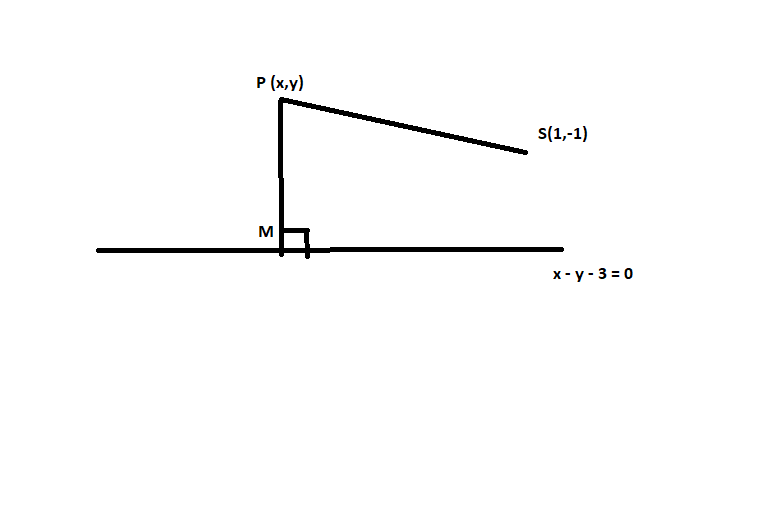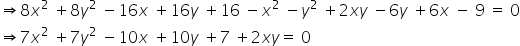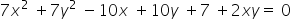Maths-
General
Easy

Question

# The equation of the ellipse whose focus is (1, -1). directrix x – y – 3 = 0 and eccentricityis

## 7x2 + 2xy + 7y2 – 10x + 10y + 7 = 0    7x2 + 2xy + 7y2 = 7 = 0    7x2 + 2xy + 7y2 + 10x – 10y – 7 = 0    none of these.Hint:

## The correct answer is: 7x2 + 2xy + 7y2 – 10x + 10y + 7 = 0

###We know that The eccentricity of ellipse, e = c/a, where c is the focal length and a is length of the semi-major axis.Thus, the equation of the ellipse whose focus is (1, -1). directrix x – y – 3 = 0 and eccentricityis#### With Turito Foundation.#### Get an Expert Advice From Turito.